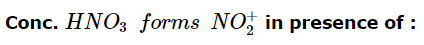Courses

# Test: Chemistry Mock- 3

## 45 Questions MCQ Test NEET Mock Test Series | Test: Chemistry Mock- 3

Description
This mock test of Test: Chemistry Mock- 3 for NEET helps you for every NEET entrance exam. This contains 45 Multiple Choice Questions for NEET Test: Chemistry Mock- 3 (mcq) to study with solutions a complete question bank. The solved questions answers in this Test: Chemistry Mock- 3 quiz give you a good mix of easy questions and tough questions. NEET students definitely take this Test: Chemistry Mock- 3 exercise for a better result in the exam. You can find other Test: Chemistry Mock- 3 extra questions, long questions & short questions for NEET on EduRev as well by searching above.
QUESTION: 1

Solution:
QUESTION: 2

Solution:
QUESTION: 3

### The reacting ion in the nitration of benzene is

Solution:
QUESTION: 4
The significant figures in 3400 are
Solution:
QUESTION: 5

Which of the following has maximum unpaired d-electrons?

Solution:
QUESTION: 6
phospholipids are esters of glycerol with
Solution:
QUESTION: 7
The shape of IF7 molecule is
Solution:
QUESTION: 8
If ammonium chloride is dissolved in water, the solution becomes cold. The change is
Solution:
QUESTION: 9
when a catalyst is added to a reversible reaction in equilibrium state, the value of equilibrium constant
Solution:
QUESTION: 10

A Carnot engine receives heat at 227oC and exhausts at 47oC. If its efficiency is to be increased by 5 % then the engine should receive heat at

Solution:
QUESTION: 11
A zero order reaction is one whose rate is independent of
Solution:
QUESTION: 12
Detergents are prepared by the action of H₂SO₄ followed by neutralization by starting with
Solution:
QUESTION: 13
The number of optical isomers of the compound CH₃CHBrCHBrCOOH is
Solution:
QUESTION: 14

Which one of the following would you expect to have highest electronegativity?

Solution:

The electronegativity values for the representative elements increase along a period from left to right. Sulphur is situated at the left of the periodic table. Thus it has highest electronegativity among the given elements. The order of electronegativity:
Mg (1.2) < B (2.0) < Te (2.01) < S (2.5) .

QUESTION: 15
The number of unpaired electrons in Ni(CO)₄ is
Solution:
QUESTION: 16
Cerium (Z=58) is an important member of lanthanoids. which of the following statement about cerium is incorrect?
Solution:
QUESTION: 17

In the reaction
Cu(s) + 2Ag⁺(aq) → Cu2⁺(aq) + 2Ag(s),
the reduction half cell reaction is

Solution:
QUESTION: 18
Zone refining process is used for the
Solution:
QUESTION: 19
In a closed flask of 5 litres, 1.0 gm H₂ is heated from 300 to 600 K, which statement is not correct ?
Solution:
QUESTION: 20

When CO is passed over solid NaOH heated to 200oC, it forms

Solution:
QUESTION: 21
Which one of the following is used to remove silicon dioxide in the Serpeck's process of purification of bauxite?
Solution:
QUESTION: 22

The structure and hybridization of Si(CH₃)₄ is

Solution:
QUESTION: 23
Among the following oxides, the least acidic is
Solution:
QUESTION: 24
By which of the following SO₂ is formed ?
Solution:
QUESTION: 25
Which one of the following can be purified by sublimation?
Solution:
QUESTION: 26

Bleaching action of bleaching powder is due to the liberation of

Solution:

On account of the formation of nascent oxygen, it shows oxidising and bleaching properties.

2CaOCl2​+H2​SO4​⟶CaCl2​+CaSO4​+2HClO

HClO⟶HCl+(O)

Coloured Matter +(O)⟶ Colourless product

Hence, option C is correct.

QUESTION: 27
Benzene is obtained by fractional distillation of
Solution:
QUESTION: 28
Hydrogen from HCl can be prepared by
Solution:
QUESTION: 29
Which of the following is a strong electrolyte?
Solution:
QUESTION: 30
A compound whose molecule is superimposable on its mirror image inspite of the presence of chiral centres, is called
Solution:
QUESTION: 31

CO₂ on reaction with ethyl magnesium bromide gives

Solution:

Answer:

Ethyl bromide does not react with CO2 but Ethyl Magnesium Bromide (Grignard Reagent) does.

CH3CH2MgBr + CO2 (dry ice) ⇒ CH3CH2COOH (acetic acid)

in presence of ether

Explanation:

Ethyl magnesium bromide (grignard reagent) reacts with solid CO2 (dry ice) in ether solvent, to form acetic acid.

QUESTION: 32
Which of the following compounds gives blood red coloration when its Lassaigne's extract is treated with alkali and ferric chloride?
Solution:
QUESTION: 33
Rosenmund's reduction of an acyl chlroide gives
Solution:
QUESTION: 34Solution:
QUESTION: 35
Which of the following compound turns black on addition of NH₄OH?
Solution:
QUESTION: 36
All the elements in a Group in the Periodic table have the same
Solution: In the modern periodic table,there are eighteen vertical columns called groups. Each group consists of a number of elements having the same number of electrons in outer shell and the same electronic configuration of the outermost shell (valence shell).
QUESTION: 37
The product of addition polymerization reaction is
Solution:
QUESTION: 38

An organic compound having molar mass 60 g mol⁻1 found to contain C = 20%,H = 6.67% and N = 46.67% ,rest being oxygen.on heating it gives NH₃ along with solid residue.The solid residue gives violet colour with alkaline copper sulphate solution.The compound is

Solution:
QUESTION: 39

Oxidation number of S in S₂O₃2⁻ is

Solution:
QUESTION: 40
Coordination number for Cu is
Solution: Coordination number of ccp (cubic close packing) arrangement is 12
Copper (cu) has ccp structure hence coordination no is 12
QUESTION: 41
How much water is needed to dilute 10 ml of 10 N hydrochloric acid to make it exactly decinormal (0.1N) ?
Solution:
QUESTION: 42
The conversion of d-form of an optically active compound into l-form of the same or of different compound or vice-versa is known as:
Solution:
QUESTION: 43
Sulphur forms the chlorides S2Cl2 and SCl2. The equivalent mass of sulphur in SCl2 is 16 g/mole. Therefore the equivalent mass of sulphur in S2Cl2 is
Solution:
QUESTION: 44
In the hydrogenation of oils the catalyst used is
Solution:
QUESTION: 45
Which one of the following pairs of substances on reaction will not evolve H₂ gas?
Solution: